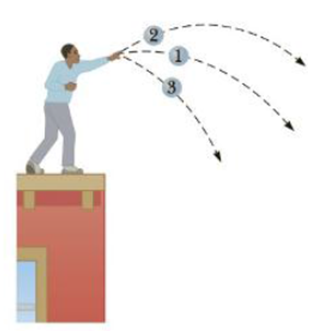Chapter 5.3, Problem 5.3QQ

Chapter
Section
Textbook Problem

Three identical halls are thrown from the top of a building, all with the same initial speed. The first ball is thrown horizontally, the second at some angle above the horizontal, and the third at some angle below the horizontal, as in Figure 5.16. Neglecting air resistance, rank the speeds of the balls as they reach the ground, from fastest to slowest. (a) 1, 2, 3 (b) 2, 1, 3 (c) 3, 1, 2 (d) All three balls strike the ground at the same speed.Figure 5.16 (Quick Quiz 5.3) A student throws three identical balls from the top of a building, each at the same initial speed hut at a different initial angle.

To determine
The speed of the balls as they reach the ground.

Explanation

Given Info:

The three balls are identical.

All three balls are having same initial speed.

All three balls are throwing from same height.

Since, all three balls are having same initial speed; the initial kinetic energy of the three balls will be the same. Since, the balls are thrown from a same height; the change in gravitational potential energy is same for all the balls. Therefore all the three balls strike the ground at same speed.

Conclusion:

Since, the initial kinetic energy and the change in gravitational potential of all the three balls are same; all the three balls strike the ground at the same speed...

Still sussing out bartleby?

Check out a sample textbook solution.

See a sample solution

The Solution to Your Study Problems

Bartleby provides explanations to thousands of textbook problems written by our experts, many with advanced degrees!

Get Started

Find more solutions based on key concepts